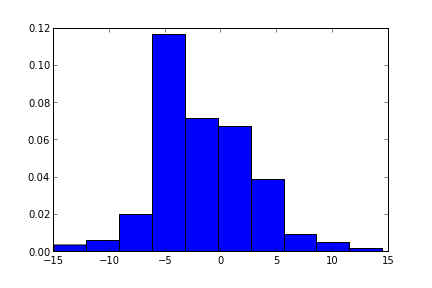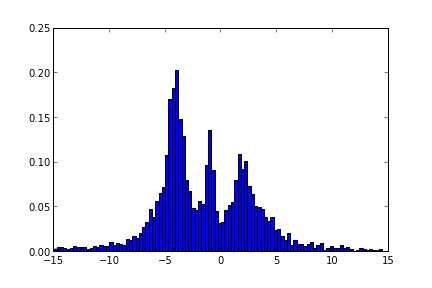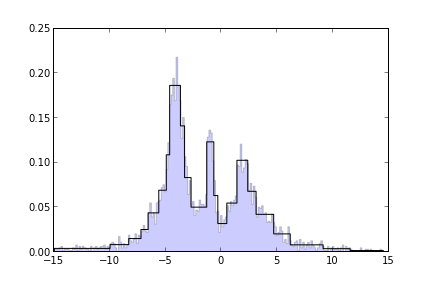# Dynamic Programming in Python: Bayesian Blocks

Of all the programming styles I have learned, dynamic programming is perhaps the most beautiful. It can take problems that, at first glance, look ugly and intractable, and solve the problem with clean, concise code. Where a simplistic algorithm might accomplish something by brute force, dynamic programming steps back, breaks the task into a smaller set of sequential parts, and then proceeds in the most efficient way possible.

### Bayesian Blocks

I'll go through an example here where the ideas of dynamic programming are vital to some very cool data analysis resuts. This post draws heavily from a recent paper by Jeff Scargle and collaborators (this is the Scargle of Lomb-Scargle Periodogram fame), as well as some conversations I had with Jeff at Astroinformatics 2012. The paper discusses a framework called Bayesian Blocks, which is essentially a method of creating histograms with bin sizes that adapt to the data (there's a bit more to it than that: here we'll focus on histograms for simplicity).

To motivate this, let's take a look at the histogram of some sampled data. We'll create a complicated set of random data in the following way:

# Define our test distribution: a mix of Cauchy-distributed variables
import numpy as np
from scipy import stats

np.random.seed(0)
x = np.concatenate([stats.cauchy(-5, 1.8).rvs(500),
stats.cauchy(-4, 0.8).rvs(2000),
stats.cauchy(-1, 0.3).rvs(500),
stats.cauchy(2, 0.8).rvs(1000),
stats.cauchy(4, 1.5).rvs(500)])

# truncate values to a reasonable range
x = x[(x > -15) & (x < 15)]


Now what does this distribution look like? We can plot a histogram to find out:

import pylab as pl
pl.hist(x, normed=True)Not too informative. The default bins in matplotlib are too wide for this dataset. We might be able to do better by increasing the number of bins:

pl.hist(x, bins=100, normed=True)This is better. But having to choose the bin width each time we plot a distribution is not only tiresome, it may lead to missing some important information in our data. In a perfect world, we'd like for the bin width to be learned in an automated fashion, based on the properties of the data itself. There have been many rules-of-thumb proposed for this task (look up Scott's Rule, Knuth's Rule, the Freedman-Diaconis Rule, and others in your favorite statistics text). But all these rules of thumb share a disadvantage: they make the assumption that all the bins are the same size. This is not necessarily optimal. But can we do better?

Scargle and collaborators showed that the answer is yes. This is their insight: For a set of histogram bins or blocks, each of an arbitrary size, one can use a Bayesian likelihood framework to compute a fitness function which only depends on two numbers: the width of each block, and the number of points in each block. The edges between these blocks (the change-points) can be varied, and the overall block configuration with the maximum fitness is quantitatively the best binning.

Simple, right?

Well, no. The problem is, as the number of points N grows large, the number of possible configurations grows as $2^N$. For N=300 points, there are already more possible configurations than the number of subatomic particles in the observable universe! Clearly an exhaustive search will fail in cases of interest. This is where dynamic programming comes to the rescue.

### Dynamic Programming

Dynamic programming is very similar to mathematical proof by induction. By way of example, consider the formula

$$1 + 2 + \cdots + n = \frac{n(n+1)}{2}.$$

How could you prove that this is true for all positive integers $n$? An inductive proof of this formula proceeds in the following fashion:

1. Base Case: We can easily show that the formula holds for $n = 1$.

2. Inductive Step: For some value $k$, assume that $1 + 2 + \cdots + k = \frac{k(k+1)}{2}$ holds. Adding $(k + 1)$ to each side and rearranging the result yields $1 + 2 + \cdots + k + (k + 1) = \frac{(k + 1)(k + 2)}{2}$. Looking closely at this, we see that we have shown the following: if our formula is true for $k$, then it must be true for $k + 1$.

3. By 1 and 2, we can show that the formula is true for any positive integer $n$, simply by starting at $n=1$ and repeating the inductive step $n - 1$ times.

Dynamic programming proceeds in much the same vein. In our Bayesian Blocks example, we can easily find the optimal binning for a single point. By making use of some mathematical proofs concerning the fitness functions, we can devise a simple step from the optimal binning for $k$ points to the optimal binning for $k + 1$ points (the details can be found in the appendices of the Scargle paper). In this way, Scargle and collaborators showed that the $2^N$ possible states can be explored in $N^2$ time.

### The Algorithm

The resulting algorithm is deceptively simple, but it can be proven to converge to the single best configuration among the $2^N$ possibilities. Below is the basic code written in python. Note that there are a few details that are missing from this version (e.g. priors on the number of bins, other forms of fitness functions, etc.) but this gets the basic job done:

def bayesian_blocks(t):
"""Bayesian Blocks Implementation

Based on algorithm outlined in http://adsabs.harvard.edu/abs/2012arXiv1207.5578S

Parameters
----------
t : ndarray, length N
data to be histogrammed

Returns
-------
bins : ndarray
array containing the (N+1) bin edges

Notes
-----
This is an incomplete implementation: it may fail for some
datasets.  Alternate fitness functions and prior forms can
be found in the paper listed above.
"""
# copy and sort the array
t = np.sort(t)
N = t.size

# create length-(N + 1) array of cell edges
edges = np.concatenate([t[:1],
0.5 * (t[1:] + t[:-1]),
t[-1:]])
block_length = t[-1] - edges

# arrays needed for the iteration
nn_vec = np.ones(N)
best = np.zeros(N, dtype=float)
last = np.zeros(N, dtype=int)

#-----------------------------------------------------------------
#-----------------------------------------------------------------
for K in range(N):
# Compute the width and count of the final bin for all possible
# locations of the K^th changepoint
width = block_length[:K + 1] - block_length[K + 1]
count_vec = np.cumsum(nn_vec[:K + 1][::-1])[::-1]

# evaluate fitness function for these possibilities
fit_vec = count_vec * (np.log(count_vec) - np.log(width))
fit_vec -= 4  # 4 comes from the prior on the number of changepoints
fit_vec[1:] += best[:K]

# find the max of the fitness: this is the K^th changepoint
i_max = np.argmax(fit_vec)
last[K] = i_max
best[K] = fit_vec[i_max]

#-----------------------------------------------------------------
# Recover changepoints by iteratively peeling off the last block
#-----------------------------------------------------------------
change_points =  np.zeros(N, dtype=int)
i_cp = N
ind = N
while True:
i_cp -= 1
change_points[i_cp] = ind
if ind == 0:
break
ind = last[ind - 1]
change_points = change_points[i_cp:]

return edges[change_points]


The details of the step from $K$ to $K + 1$ may be a bit confusing from this implementation: it boils down to the fact that Scargle et al. were able to show that given an optimal configuration of $K$ points, the $(K + 1)$^th configuration is limited to one of $K$ possibilities.

The function as written above takes a sequence of points, and returns the edges of the optimal bins. We'll visualize the result on top of the histogram we saw earlier:

# plot a standard histogram in the background, with alpha transparency
H1 = hist(x, bins=200, histtype='stepfilled',
alpha=0.2, normed=True)
# plot an adaptive-width histogram on top
H2 = hist(x, bins=bayesian_blocks(x), color='black',
histtype='step', normed=True)The adaptive-width bins lead to a very clean representation of the important features in the data. More importantly, these bins are quantifiably optimal, and their properties can be used to make quantitative statistical statements about the nature of the data. This type of procedure has proven very useful in analysis of time-series data in Astronomy.

### Conclusion

We've just scratched the surface of Bayesian Blocks and Dynamic Programming. Some of the more interesting details of this algorithm require much more depth: the appendicies of the Scargle paper provide these details. Dynamic Programming ideas have been shown to be useful in many optimization problems. One other example I've worked with extensively is Dijkstra's Algorithm for computing the shortest paths on a connected graph. This is available in the scipy.sparse.csgraph submodule, which is included in the most recent release of scipy.

The above python implementation of Bayesian Blocks is an extremely basic form of the algorithm: I plan to include some more sophisticated options in the python package I'm currently working on, called astroML: Machine Learning for Astrophysics. I'll release version 0.1 of astroML at the end of October 2012, in time to present it at CIDU 2012. If you're interested, I'll have updates here on the blog, as well as on my twitter feed.

Update: astroML version 0.1 has been released: see the web site here. It includes a full-featured Bayesian blocks implementation with histogram tools, which you can read about here.

Finally, all of the above code snippets are available as an ipython notebook: bayesian_blocks.ipynb. For information on how to view this file, see the IPython page. Alternatively, you can view this notebook (but not modify it) using the nbviewer utility here.